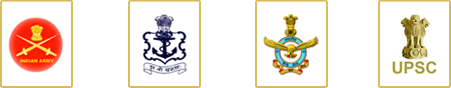### 5. Differential Calculus

Concept of a real valued function – domain, range and graph of a function. Composite functions, one to one, onto and inverse functions. Notion of limit, Standard limits – examples. Continuity of functions – examples, algebraic operations on continuous functions. Derivative of a function at a point, geometrical and physical interpreatation of a derivative – applications. Derivatives of sum, product and quotient of functions, derivative of a function with respect of another function, derivative of a composite function. Second order derivatives. Increasing and decreasing functions. Application of derivatives in problems of maxima and minima.

### Important Information